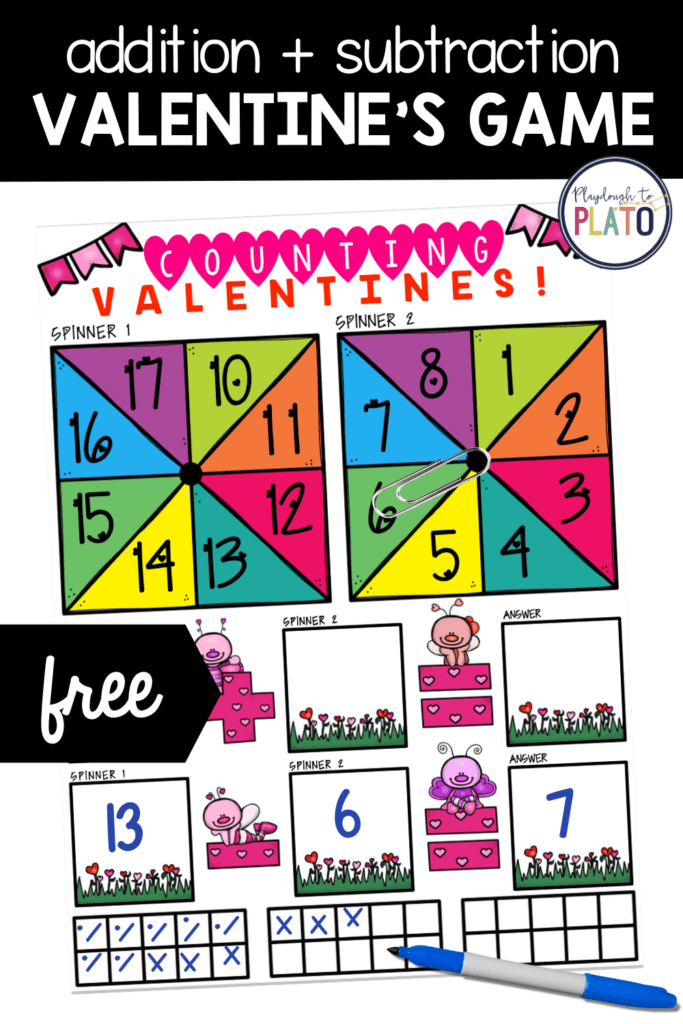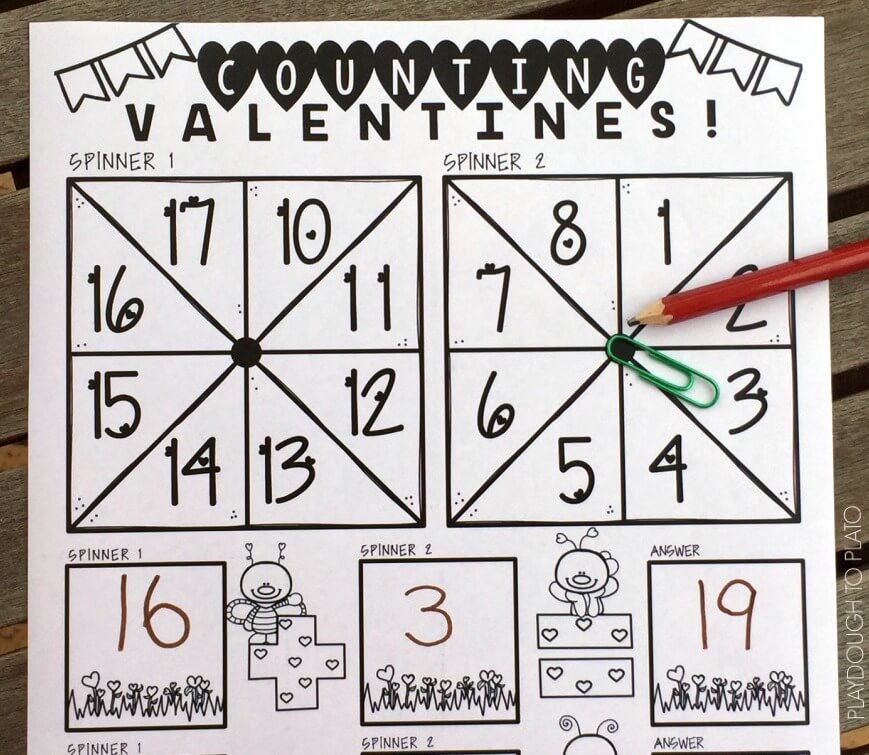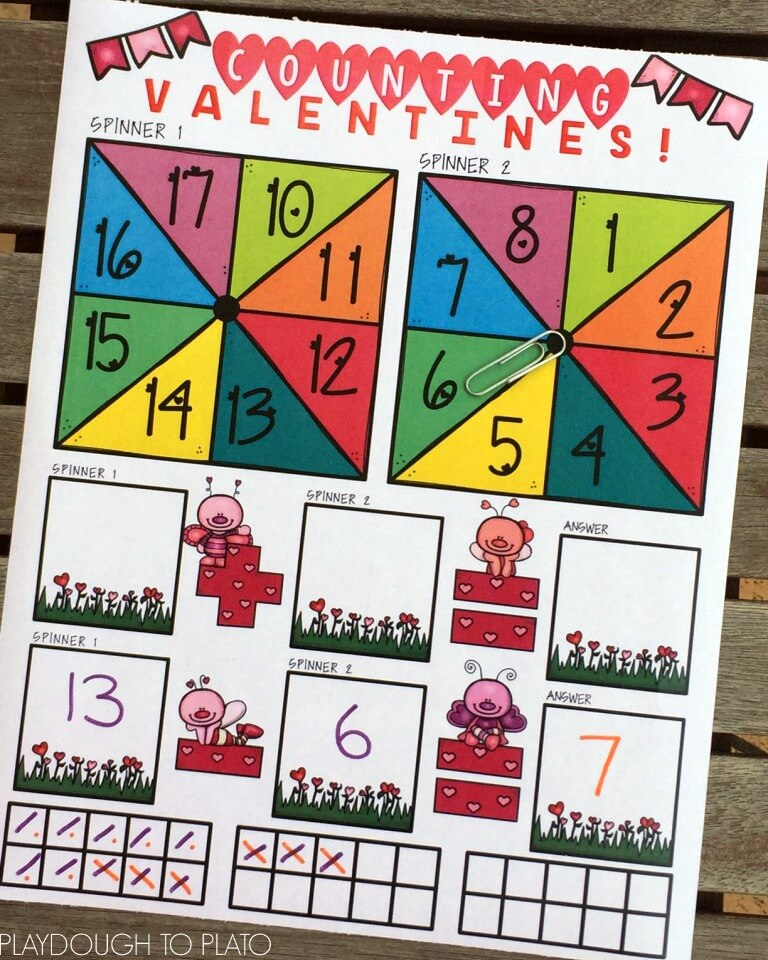# Valentine’s Addition and Subtraction Math Game

Valentine’s Day is just around the corner, so some thematic learning is in order with a Valentine’s math game! Practice counting valentines with these addition and subtraction re-usable mats. They focus mainly on numbers to 20, so they’re perfect for first grade but are manageable for kindergarteners, too.

It’s the perfect complement to our popular Place Value Cover Up!

This post contains Amazon affiliate links.I downloaded the counting valentines mats (below), printed and laminated them and then grabbed pencils and paper clips for spinning and some dry erase markers that could be wiped clean after use.

If you have some spare brads (like these ones) it would be worthwhile attaching them to the spinners as they’re easier for kids to use than the pencil and paper clip method.After reminding the kids how to put a paper clip around the tip of a pencil at the center dot of the spinner, and having a few practices at flicking the paper clip to find a random number, the children split into pairs.

One child spun the first spinner and wrote the number into the first square of the addition equation (labeled “spinner 1”). The second child did the same with the second spinner, writing the answer in the second square of the addition equation (“labeled ‘spinner 2”).

Most of the children then used mental math (and fingers) to add the two numbers and wrote the answer in the third “answer” square. To check their answer, they made marks in the ten frames at the bottom of the page to represent the two numbers from the spinners, then counted how many.

Some children were not comfortable with doing addition in their head, so they went straight to the ten frames and checked off the squares. This gave them the confidence to complete the equation independently.## Valentine’s Subtraction Math Game

After completing the addition version of the game, the children spun the spinners again and wrote those two numbers into the first two squares of the subtraction equation.

They then used mental math and fingers to subtract the second number from the first and wrote the answer in the third square.

More children had difficulty with the subtraction and relied more heavily on the ten frames to be sure of their answer.

They marked squares for the first number in the equation and then used another color or a different kind of mark to work backwards, crossing off the number of squares represented by the second number in the equation.

Finally, they counted up the remaining squares to determine or double check the answer.

Once they had successfully answered both equations, students used a tissue to wipe the laminated mat clean and started over.